PA 1.

LO 10.1When prices are rising (inflation), which costing method would produce the highest value for gross margin? Choose between first-in, first-out (FIFO); last-in, first-out (LIFO); and weighted average (AVG).

Evansville Company had the following transactions for the month.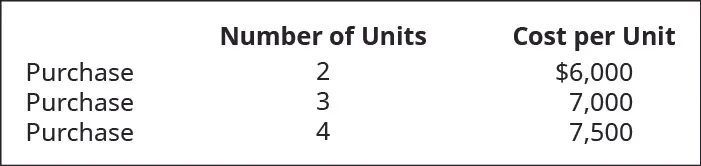Calculate the gross margin for each of the following cost allocation methods, assuming A62 sold just one unit of these goods for $10,000. Provide your calculations. 1. first-in, first-out (FIFO) 2. last-in, first-out (LIFO) 3. weighted average (AVG) PA 2. LO 10.2Trini Company had the following transactions for the month.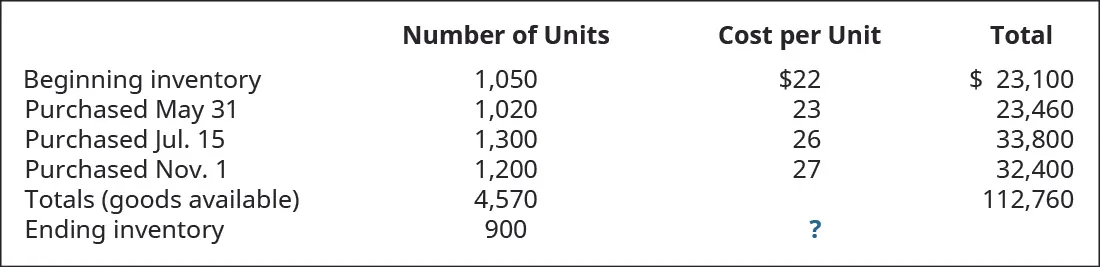Calculate the ending inventory dollar value for each of the following cost allocation methods, using periodic inventory updating. Provide your calculations. 1. first-in, first-out (FIFO) 2. last-in, first-out (LIFO) 3. weighted average (AVG) PA 3. LO 10.2Trini Company had the following transactions for the month.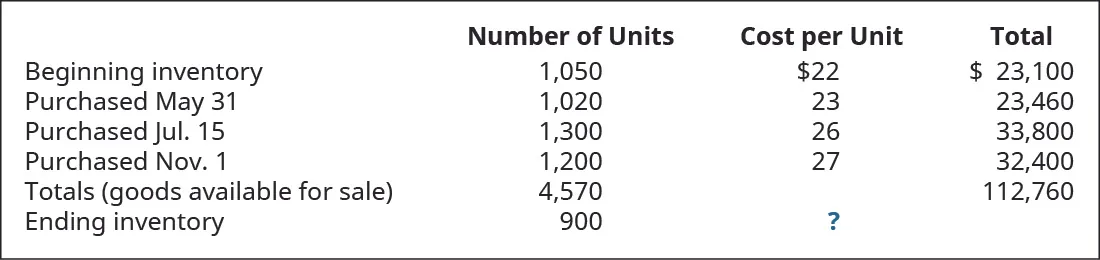Calculate the cost of goods sold dollar value for the period for each of the following cost allocation methods, using periodic inventory updating. Provide your calculations. 1. first-in, first-out (FIFO) 2. last-in, first-out (LIFO) 3. weighted average (AVG) PA 4. LO 10.3Calculate the cost of goods sold dollar value for A74 Company for the sale on March 11, considering the following transactions under three different cost allocation methods and using perpetual inventory updating. Provide calculations for (a) first-in, first-out (FIFO); (b) last-in, first-out (LIFO); and (c) weighted average (AVG).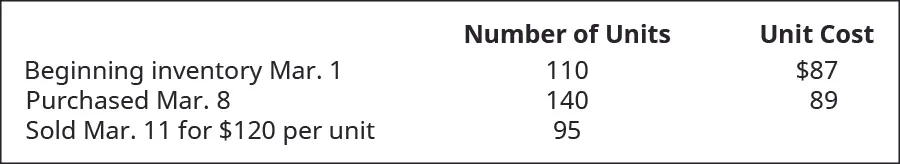PA 5. LO 10.3Use the first-in, first-out (FIFO) cost allocation method, with perpetual inventory updating, to calculate (a) sales revenue, (b) cost of goods sold, and c) gross margin for A75 Company, considering the following transactions.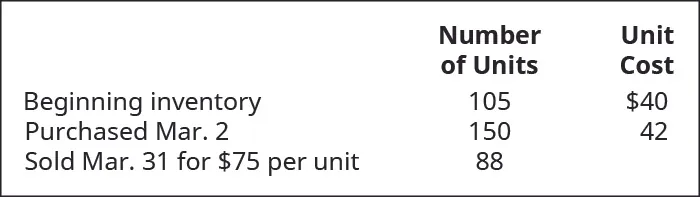PA 6. LO 10.3Use the last-in, first-out (LIFO) cost allocation method, with perpetual inventory updating, to calculate (a) sales revenue, (b) cost of goods sold, and c) gross margin for A75 Company, considering the following transactions.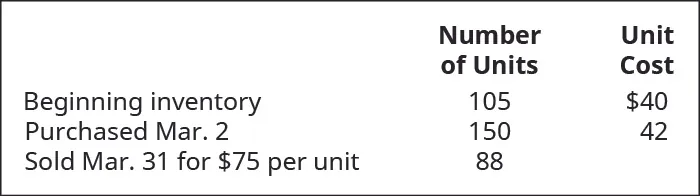PA 7. LO 10.3Use the weighted-average (AVG) cost allocation method, with perpetual inventory updating, to calculate (a) sales revenue, (b) cost of goods sold, and c) gross margin for A75 Company, considering the following transactions.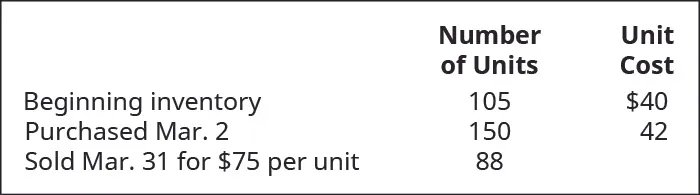PA 8. LO 10.3Prepare journal entries to record the following transactions, assuming perpetual inventory updating and first-in, first-out (FIFO) cost allocation. Assume no beginning inventory.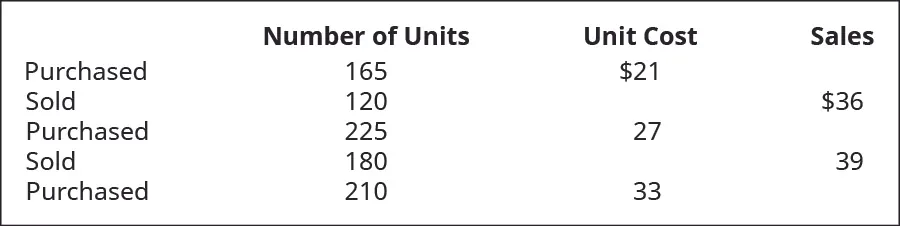PA 9. LO 10.3Calculate a) cost of goods sold, b) ending inventory, and c) gross margin for A76 Company, considering the following transactions under three different cost allocation methods and using perpetual inventory updating. Provide calculations for first-in, first-out (FIFO).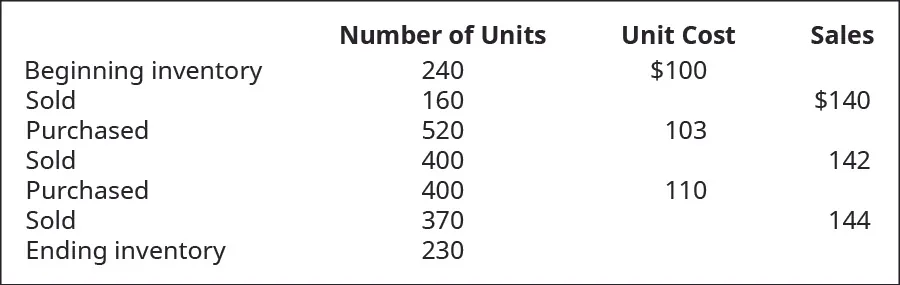PA 10. LO 10.3Calculate a) cost of goods sold, b) ending inventory, and c) gross margin for A76 Company, considering the following transactions under three different cost allocation methods and using perpetual inventory updating. Provide calculations for last-in, first-out (LIFO).PA 11. LO 10.3Calculate a) cost of goods sold, b) ending inventory, and c) gross margin for A76 Company, considering the following transactions under three different cost allocation methods and using perpetual inventory updating. Provide calculations for weighted average (AVG).PA 12. LO 10.3Compare the calculations for gross margin for A76 Company, based on the results of the perpetual inventory calculations using FIFO, LIFO, and AVG. PA 13. LO 10.4Company Elmira reported the following cost of goods sold but later realized that an error had been made in ending inventory for year 2021. The correct inventory amount for 2021 was 32,000. Once the error is corrected, (a) how much is the restated cost of goods sold for 2021? and (b) how much is the restated cost of goods sold for 2022?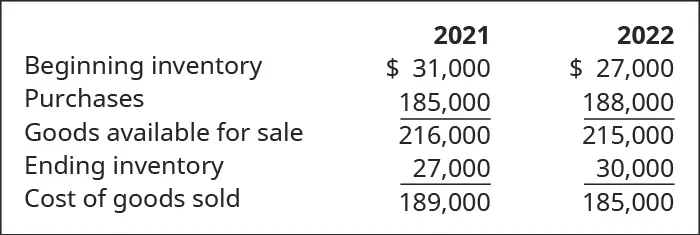PA 14. LO 10.4Assuming a company’s year-end inventory were overstated by$5,000, indicate the effect (overstated/understated/no effect) of the error on the following balance sheet and income statement accounts.

1. Income Statement: Cost of Goods Sold
2. Income Statement: Net Income
3. Balance Sheet: Assets
4. Balance Sheet: Liabilities
5. Balance Sheet: Equity
PA 15.

LO 10.5Use the following information relating to Shana Company to calculate the inventory turnover ratio and the number of days’ sales in inventory ratio.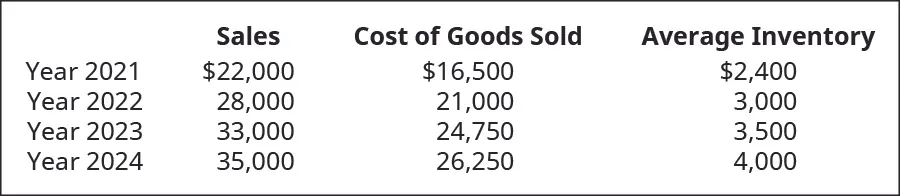PA 16.

LO 10.5Use the following information relating to Clover Company to calculate the inventory turnover ratio, gross margin, and the number of days’ sales in inventory ratio, for years 2022 and 2023.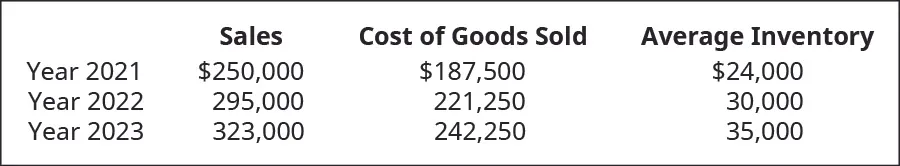Order a print copy

As an Amazon Associate we earn from qualifying purchases.